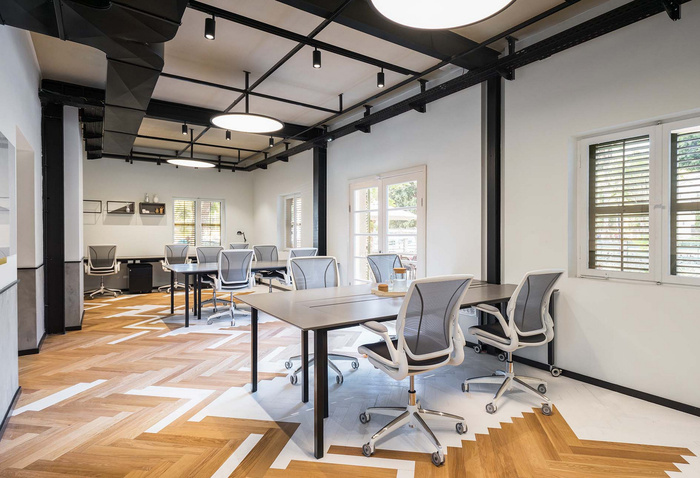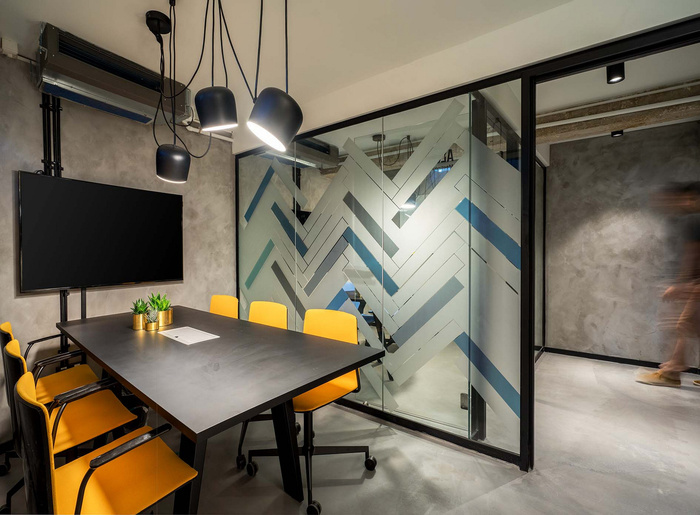|

# 蒂凡利普办公家具——浅谈家具色彩搭配

讲解色彩的搭配有哪些：现代办公家具厂大概都有5种色调：黑色、灰色、棕色、暗红和素蓝色。通常，不同种类的灰色用于办公桌；黑、棕色用于大班椅和会议椅；素蓝和暗红多作职员椅。因为，蓝色虽淡雅，不失明快；红色虽庄重，不乏活泼；黑、棕色向来给人凝重感，用它们装点会议桌，会促使你集中思考一般来说，办公室色彩的配置要依照“大跳跃，小和谐”的原则。大跳跃是指办公室之间的色彩变化。比如，你有3间办公室，3间屋子可选择完全不同的主调，但每间屋的门窗、桌椅和地板甚至明面的办公室档案资料柜和琐碎的办公用品都要保持自己的整体和谐，就是小和谐的原则。这样，尽管你的工作活动范围有限，每到一处都会给你耳目一新的感觉。在办公室中，每一种色彩都有它自己的语言，它会向你的同事和客户传达出一定的心理讯息。比如，黑色给人孤独感，但同时也有一种高贵和庄重；棕色让人觉得老气横秋，但不同浓度的棕不但没有了年老的晦暗，还会产生出几分优雅；大红大粉过于张扬，若和安静的冷色搭调，能够显出年轻的活泼；本白土黄过分素净，若和快乐的暖色牵手，就易于露出自己的典雅。再进一步，淡紫配天蓝给人恬静的感觉；洋红配宝蓝让人觉得明快；酱紫配月白则显得高雅；粉红配本白传达青春气息；深棕配浅黄可以让你看起来比较成熟；浅灰配墨黑显得庄重；朱红配黑能吸引对方的视线；墨绿配土黄的搭配最自然；海蓝配浅蓝则令人感觉踏实；至于明黄配墨黑，则可以给人跳跃的美。

`声明：本文由入驻焦点开放平台的作者撰写，除焦点官方账号外，观点仅代表作者本人，不代表焦点立场错误信息举报电话： 400-099-0099，邮箱：jubao@vip.sohu.com，或点此进行意见反馈，或点此进行举报投诉。`A B C D E F G H J K L M N P Q R S T W X Y Z
A - B - C - D - E
• A
• 鞍山
• 安庆
• 安阳
• 安顺
• 安康
• 澳门
• B
• 北京
• 保定
• 包头
• 巴彦淖尔
• 本溪
• 蚌埠
• 亳州
• 滨州
• 北海
• 百色
• 巴中
• 毕节
• 保山
• 宝鸡
• 白银
• 巴州
• C
• 承德
• 沧州
• 长治
• 赤峰
• 朝阳
• 长春
• 常州
• 滁州
• 池州
• 长沙
• 常德
• 郴州
• 潮州
• 崇左
• 重庆
• 成都
• 楚雄
• 昌都
• 慈溪
• 常熟
• D
• 大同
• 大连
• 丹东
• 大庆
• 东营
• 德州
• 东莞
• 德阳
• 达州
• 大理
• 德宏
• 定西
• 儋州
• 东平
• E
• 鄂尔多斯
• 鄂州
• 恩施
F - G - H - I - J
• F
• 抚顺
• 阜新
• 阜阳
• 福州
• 抚州
• 佛山
• 防城港
• G
• 赣州
• 广州
• 桂林
• 贵港
• 广元
• 广安
• 贵阳
• 固原
• H
• 邯郸
• 衡水
• 呼和浩特
• 呼伦贝尔
• 葫芦岛
• 哈尔滨
• 黑河
• 淮安
• 杭州
• 湖州
• 合肥
• 淮南
• 淮北
• 黄山
• 菏泽
• 鹤壁
• 黄石
• 黄冈
• 衡阳
• 怀化
• 惠州
• 河源
• 贺州
• 河池
• 海口
• 红河
• 汉中
• 海东
• 怀来
• I
• J
• 晋中
• 锦州
• 吉林
• 鸡西
• 佳木斯
• 嘉兴
• 金华
• 景德镇
• 九江
• 吉安
• 济南
• 济宁
• 焦作
• 荆门
• 荆州
• 江门
• 揭阳
• 金昌
• 酒泉
• 嘉峪关
K - L - M - N - P
• K
• 开封
• 昆明
• 昆山
• L
• 廊坊
• 临汾
• 辽阳
• 连云港
• 丽水
• 六安
• 龙岩
• 莱芜
• 临沂
• 聊城
• 洛阳
• 漯河
• 娄底
• 柳州
• 来宾
• 泸州
• 乐山
• 六盘水
• 丽江
• 临沧
• 拉萨
• 林芝
• 兰州
• 陇南
• M
• 牡丹江
• 马鞍山
• 茂名
• 梅州
• 绵阳
• 眉山
• N
• 南京
• 南通
• 宁波
• 南平
• 宁德
• 南昌
• 南阳
• 南宁
• 内江
• 南充
• P
• 盘锦
• 莆田
• 平顶山
• 濮阳
• 攀枝花
• 普洱
• 平凉
Q - R - S - T - W
• Q
• 秦皇岛
• 齐齐哈尔
• 衢州
• 泉州
• 青岛
• 清远
• 钦州
• 黔南
• 曲靖
• 庆阳
• R
• 日照
• 日喀则
• S
• 石家庄
• 沈阳
• 双鸭山
• 绥化
• 上海
• 苏州
• 宿迁
• 绍兴
• 宿州
• 三明
• 上饶
• 三门峡
• 商丘
• 十堰
• 随州
• 邵阳
• 韶关
• 深圳
• 汕头
• 汕尾
• 三亚
• 三沙
• 遂宁
• 山南
• 商洛
• 石嘴山
• T
• 天津
• 唐山
• 太原
• 通辽
• 铁岭
• 泰州
• 台州
• 铜陵
• 泰安
• 铜仁
• 铜川
• 天水
• 天门
• W
• 乌海
• 乌兰察布
• 无锡
• 温州
• 芜湖
• 潍坊
• 威海
• 武汉
• 梧州
• 渭南
• 武威
• 吴忠
• 乌鲁木齐
X - Y - Z
• X
• 邢台
• 徐州
• 宣城
• 厦门
• 新乡
• 许昌
• 信阳
• 襄阳
• 孝感
• 咸宁
• 湘潭
• 湘西
• 西双版纳
• 西安
• 咸阳
• 西宁
• 仙桃
• 西昌
• Y
• 运城
• 营口
• 盐城
• 扬州
• 鹰潭
• 宜春
• 烟台
• 宜昌
• 岳阳
• 益阳
• 永州
• 阳江
• 云浮
• 玉林
• 宜宾
• 雅安
• 玉溪
• 延安
• 榆林
• 银川
• Z
• 张家口
• 镇江
• 舟山
• 漳州
• 淄博
• 枣庄
• 郑州
• 周口
• 驻马店
• 株洲
• 张家界
• 珠海
• 湛江
• 肇庆
• 中山
• 自贡
• 资阳
• 遵义
• 昭通
• 张掖
• 中卫

1室1厅1厨1卫1阳台

1
2
3
4
5

0
1
2

1

1

0
1
2
3报名成功，资料已提交审核A B C D E F G H J K L M N P Q R S T W X Y Z
A - B - C - D - E
• A
• 鞍山
• 安庆
• 安阳
• 安顺
• 安康
• 澳门
• B
• 北京
• 保定
• 包头
• 巴彦淖尔
• 本溪
• 蚌埠
• 亳州
• 滨州
• 北海
• 百色
• 巴中
• 毕节
• 保山
• 宝鸡
• 白银
• 巴州
• C
• 承德
• 沧州
• 长治
• 赤峰
• 朝阳
• 长春
• 常州
• 滁州
• 池州
• 长沙
• 常德
• 郴州
• 潮州
• 崇左
• 重庆
• 成都
• 楚雄
• 昌都
• 慈溪
• 常熟
• D
• 大同
• 大连
• 丹东
• 大庆
• 东营
• 德州
• 东莞
• 德阳
• 达州
• 大理
• 德宏
• 定西
• 儋州
• 东平
• E
• 鄂尔多斯
• 鄂州
• 恩施
F - G - H - I - J
• F
• 抚顺
• 阜新
• 阜阳
• 福州
• 抚州
• 佛山
• 防城港
• G
• 赣州
• 广州
• 桂林
• 贵港
• 广元
• 广安
• 贵阳
• 固原
• H
• 邯郸
• 衡水
• 呼和浩特
• 呼伦贝尔
• 葫芦岛
• 哈尔滨
• 黑河
• 淮安
• 杭州
• 湖州
• 合肥
• 淮南
• 淮北
• 黄山
• 菏泽
• 鹤壁
• 黄石
• 黄冈
• 衡阳
• 怀化
• 惠州
• 河源
• 贺州
• 河池
• 海口
• 红河
• 汉中
• 海东
• 怀来
• I
• J
• 晋中
• 锦州
• 吉林
• 鸡西
• 佳木斯
• 嘉兴
• 金华
• 景德镇
• 九江
• 吉安
• 济南
• 济宁
• 焦作
• 荆门
• 荆州
• 江门
• 揭阳
• 金昌
• 酒泉
• 嘉峪关
K - L - M - N - P
• K
• 开封
• 昆明
• 昆山
• L
• 廊坊
• 临汾
• 辽阳
• 连云港
• 丽水
• 六安
• 龙岩
• 莱芜
• 临沂
• 聊城
• 洛阳
• 漯河
• 娄底
• 柳州
• 来宾
• 泸州
• 乐山
• 六盘水
• 丽江
• 临沧
• 拉萨
• 林芝
• 兰州
• 陇南
• M
• 牡丹江
• 马鞍山
• 茂名
• 梅州
• 绵阳
• 眉山
• N
• 南京
• 南通
• 宁波
• 南平
• 宁德
• 南昌
• 南阳
• 南宁
• 内江
• 南充
• P
• 盘锦
• 莆田
• 平顶山
• 濮阳
• 攀枝花
• 普洱
• 平凉
Q - R - S - T - W
• Q
• 秦皇岛
• 齐齐哈尔
• 衢州
• 泉州
• 青岛
• 清远
• 钦州
• 黔南
• 曲靖
• 庆阳
• R
• 日照
• 日喀则
• S
• 石家庄
• 沈阳
• 双鸭山
• 绥化
• 上海
• 苏州
• 宿迁
• 绍兴
• 宿州
• 三明
• 上饶
• 三门峡
• 商丘
• 十堰
• 随州
• 邵阳
• 韶关
• 深圳
• 汕头
• 汕尾
• 三亚
• 三沙
• 遂宁
• 山南
• 商洛
• 石嘴山
• T
• 天津
• 唐山
• 太原
• 通辽
• 铁岭
• 泰州
• 台州
• 铜陵
• 泰安
• 铜仁
• 铜川
• 天水
• 天门
• W
• 乌海
• 乌兰察布
• 无锡
• 温州
• 芜湖
• 潍坊
• 威海
• 武汉
• 梧州
• 渭南
• 武威
• 吴忠
• 乌鲁木齐
X - Y - Z
• X
• 邢台
• 徐州
• 宣城
• 厦门
• 新乡
• 许昌
• 信阳
• 襄阳
• 孝感
• 咸宁
• 湘潭
• 湘西
• 西双版纳
• 西安
• 咸阳
• 西宁
• 仙桃
• 西昌
• Y
• 运城
• 营口
• 盐城
• 扬州
• 鹰潭
• 宜春
• 烟台
• 宜昌
• 岳阳
• 益阳
• 永州
• 阳江
• 云浮
• 玉林
• 宜宾
• 雅安
• 玉溪
• 延安
• 榆林
• 银川
• Z
• 张家口
• 镇江
• 舟山
• 漳州
• 淄博
• 枣庄
• 郑州
• 周口
• 驻马店
• 株洲
• 张家界
• 珠海
• 湛江
• 肇庆
• 中山
• 自贡
• 资阳
• 遵义
• 昭通
• 张掖
• 中卫• 手机• 分享
• 设计
免费设计
• 计算器
装修计算器
• 入驻
合作入驻
• 联系
联系我们
• 置顶
返回顶部